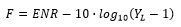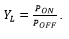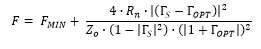Webcast Overview

# Tuners for noise parameter measurement

By combining a tuner and a PNA-X network analyzer, it is possible to set up highly efficient measuring stations for S parameter, noise figure, noise parameter and intermodulation measurements:

The noise figure of an amplifier is typically measured by means of a noise source in combination with a spectrum analyzer. Normally, the established Y factor method will be used, where a noise source at the amplifier input is switched into two states: first into the OFF state, which is an approximation of a 50Ω termination; and then into the ON state, whereby an active noise power with a defined value is created. The ENR factor ("Excessive Noise Ratio") represents the relation between the noise power density in the ON and OFF states. It is measured in [dB] and may be frequency-dependent. In practice, noise sources are implemented with a Read diode as a generator in combination with a downstream attenuator.Figure 1: Basic measuring station layout for the determination of noise parameters and the measurement of S parameters. The setup may be expanded for intermodulation measurements.

Since the complex reflection factor of the noise generator is different for each of the two switch states, it is necessary to determine the noise reflection factor of the noise source for both switch states first in order to obtain exact measurements of the noise figure. With these measurement values, it is then possible to correct the noise figure. The goal of the correction is to obtain a valid noise figure for a circuit with a source load of precisely 50Ω. If an ideal 50Ω source load is assumed, the noise figure for an ambient temperature of 20°C can be calculated in [dB] as:where the noise power density ratio measured at the amplifier output for the ON and OFF states, YL, is defined as:An alternative method for measuring noise figures is the so-called "Cold Source" method. If this method is used, it is also necessary to determine the exact value of the test amplifier gain, since this will now be included into the calculation. Therefore, it always makes sense to measure the gain and noise figure of the amplifier under test at the same time, without having to change the setup of the measuring station.Figure 2: Noise figure as a function of the source impedance of the noise source, Γ_S.

Let us now have a look at the case of a noise source with variable source impedance, which can be adjusted by means of a tuner. The ohmic losses of the tuner and feed lines and the reflection factor of the noise source are not taken into account. The noise figure of the amplifier can now be analytically represented as a function of the source impedance (Zis the characteristic wave impedance of 50Ω; Γ is the complex reflection factor of the source, Γopt is the complex reflection factor of the source for optimum noise matching, when the minimum noise figure of Fmin is achieved; Rn defines the steepness of the noise figure increments):This means that four noise parameters are required in order to describe the noise behavior of an amplifier: Fmin, Rn, the real component {Γopt} and the imaginary component {Γopt}. This formula is extremely useful for practical purposes, since it describes the increase of the noise figure in case of a mismatch. A mismatch may occur due to unsuitable matching circuits at the amplifier input, or due to tolerances of the passive components of the matching network/the active semiconductor circuit. Figure 2 shows an example noise figure distribution calculated with the formula described above. If this graphic is viewed from above (and thereby reduced to two dimensions), the Nyquist plots for constant noise figures are visible as circles around the point of optimum noise matching, Γopt.

If the ohmic losses and the reflection factor of the noise source are taken into account, the process to evaluate the noise figure becomes very elaborate. In this case, it is not sufficient to use just the Y factor method or the cold source method, so the software makes additional corrections.  This requires two calibrations with the network analyzer before performing the noise measurement: one 2-port-calibration for both reference planes of the amplifier under test and one 1-port-calibration for the reference plane of the noise source. The noise parameters are then obtained by using a fitting procedure on the measurement data.

The above photograph shows a noise measuring station which uses a network analyzer with optional internal HF switches and a vectoral noise receiver. The internal HF switches allow switching between measuring the S parameters and the noise figure. With a further option of the network analyzer, this measuring station can support additional measurements of the 2-tone intermodulation performance of the amplifier under test.

We recommend the components listed in the following table for automatic noise measuring stations which are used to obtain noise parameters:

 Noise source For amplifiers with a gain of 10-20dB, we recommend noise sources with an ENR of around 15dB. Tuners Motorized mechanical tuners are ideal. Amplifier under test The amplifier component to be tested will be contacted via a test socket or via the wafer prober. Possible measurement falsifications which are due to electromagnetic interference can be prohibited by using special shielded housings with EMC-proof cable ducts for power supply and HF cabling. Noise receivers For this purpose, highly sensitive spectrum analyzers may be used. A very efficient alternative are high-quality PNA-X network analyzers, equipped with option "vectoral noise measurement" and internal HF switches for switching between measuring the gain and the noise power. If a Keysight PNA-X is used, it is possible to integrate the 2-tone intermodulation measurement with additional options. Measurement cables As measurement cables, we recommend phase-stable, low-loss measurement cables, e.g. W.L. GORE Phaseflex. It is a basic requirement to place the noise source as closely as possible to the DUT or the tuner. However, this is not always possible when a waver prober is used, and lighter HF cables may have to be used for contacting the DUTs. HF switches at the input of the amplifier under test The best option are low-loss, reliable HF switches which can be controlled by software. Pre-amplifier at the output of the amplifier under test In many cases, the gain of the amplifier under test or the input sensitivity of the spectrum analyzer will not be sufficient to measure the noise level correctly. In these cases, an additional low-noise pre-amplifier is required. Software As controller and for obtaining the measurement data it is recommended to put together the available modules of the controller software of the tuner manufacturer.

The following examples show the measurement results for two different amplifiers under test:

• SGA4363Z: Repeater by QORVO. Well matched to 50Ω at the input and at the output.
• BFP193: Discrete HF transistor by Infineon Technologies AG. Not matched at the input; the output has an impedance of approx. 50Ω.

Both amplifiers have been mounted in a shielded metal housing and are powered by battery in order to minimize any influence by external interferences (for the measurement setup, see Figure 3).  The picture below shows how the individual components in the configuration menus of the ATSv5 SW were adapted to the available measurement instruments: